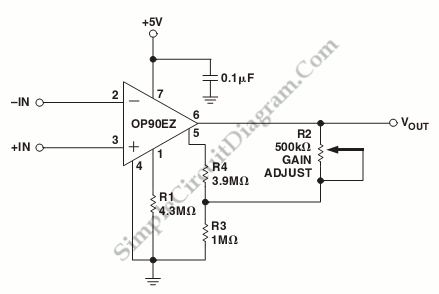# Micropower Single-Supply Instrumentation Amplifier

A schematic diagram of a micropower single-supply instrumentation amplifier circuit is shown below. This circuit only need 15µA of current and can give over 110dB of common rejection. A single amplifier can provide differential to single-ended conversion with excellent common mode-rejection because feedback is to trim pins rather than to the inverting pin. Here is the schematic diagram of the circuit:This circuit is restricted to high gain application because distortion of the instrumentation amplifier is that of a differential pair. For gains of of 500 to 1000 over a 2.5 V output range, nonlinearity of this circuit is less than 0.1%. The voltage gain is set by resistors R4 and R3, with the values shown, the voltage gain of this circuit is 1000. The instrumentation amplifier has gain tempco of 50 ppm/°C. This circuit has offset voltage of under 150 µV with drift below 2 µV/°C. This circuit can provide true “zero-in, zero-out” operation because this circuit use OP90 that has input and output range include the negative rail. [circuit’s schematic diagram source: analog.com]